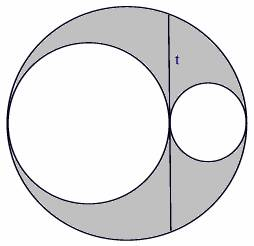favorite We need a little bit of your help to keep things running, click on this banner to learn more
Problems

In the picture below you can see two small circles touching each other. The larger circle touches both pf them. The length of the common tangent inscribed inside the larger circle is t and the radiuses of the two smaller circles are r1 and r2. The centers of the three circles are collinear. You will be given the value of r1 and r2 or the value of t. You will have to find the area that is within the larger circle but out of the two smaller circles (marked gray in the picture). If the given data is not enough to find the gray area, print the line "Impossible." in a single line.Input

The first line contains the number of test cases n (n ≤ 100). Each of the next n lines contain a set of input.

Each set either contains one or two integer. If it contains one integer then it is the value of t, otherwise the two values are the values of r1 and r2. All these integers are less than 100.

Output

For each line of input produce one line of output. This line contains the area of the gray part if the given information is enough to find the area of the gray part. Otherwise it contains the line "Impossible." The area should have four digits after the decimal point.

Time limit 1 second
Memory limit 64 MiB
Input example #1
2
10 10
15 20

Output example #1
628.3185
1884.9556